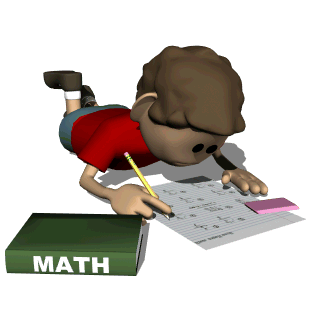•

 Ms. Hart's Learning Factory

#Learn how to solve various concepts on these sites.

Search Elementary Topics
State Testing Practice

 Problem Solving A series of situation-based, multiple-choice problems http://www.gamequarium.org/dir/Gamequarium/Math/Problem_Solving/ Word problems in game formats http://mathplayground.com/wordproblems.html

 Computation with Decimals All types of decimal operations http://www.gamequarium.org/dir/Gamequarium/Math/Decimals/ Problems involving money http://www.gamequarium.org/dir/Gamequarium/Math/Money_Math/ Game using fraction, decimals, and percents http://mathplayground.com/Decention/Decention.html More with money http://mathplayground.com/making_change.html Shopping with percents http://mathplayground.com/percent_shopping.html

 Geometry Videos explaining geometric properties http://www.gamequarium.org/dir/SqoolTube_Videos/Math/Geometry/ Problems involving geometric figures and properties http://www.gamequarium.org/dir/Gamequarium/Math/Geometry/ Geometry for 5th graders http://nlvm.usu.edu/en/nav/category_g_2_t_3.html

Math learning videos on a variety of topics:

Grade 5 Test Prep problems and skills practice:

Logic games:

Math games by grade level – all topics: